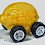## Monday, May 18, 2015

### Another mistake from Romer

I found another mistake in Romer's "proof", but I also got some (wrong-headed) pushback on the econ job rumors forum about my take on Paul Romer's mathiness. Here's the comment:
Yeah, [this post] is complete garbage. Mathematically, it's an issue of pointwise vs. uniform convergence, plain and simple. Economically, Romer says that Lucas + Moll don't get endogenous growth because of diffusion. Rather, they get endogenous growth because the "knowledge frontier" is already unbounded. Any empirical observations (which necessarily occur at finite T) depend critically on the rate of knowledge arrival. This guy just does not get it.
I think this shows us a bit where economics has gone off the rails with the mathiness. Economics should never refer to uniform vs pointwise convergence. This is a topic from real analysis . If uniform vs pointwise convergence matters in economics, you're doing it wrong.

And it's not even a correct assessment -- it's actually a case of almost uniform convergence (the set of points where the limit doesn't converge uniformly has measure zero, and is in fact exactly the point $\beta = 0$).

An example from physics might help make this a bit clearer. Let's say we have a model of all possible collections of nuclei that decay via $\alpha$ decay with time constant $\tau$ so that the number of your original nuclei left at time t is given by

$$N_{\tau}(t) = N_{0} e^{-t/\tau}$$

This function has the essential properties of Lucas and Moll's growth function $g_{\beta}(t)$ where basically $1/\beta$ is now $\tau$.

$$\lim_{\tau \rightarrow \infty} N_{\tau}(T) = 1$$

$$\lim_{t \rightarrow \infty} N_{\tau}(t) = 0$$

The English versions of these two limits:

1. If the nuclei are stable, they never decay.
2. Unstable nuclei always eventually decay.

Lucas and Moll (2014) essentially is a model of decaying nuclei and their limit is the second one. They don't care about stable nuclei (economies where nothing happens), they care about something else (economies where everything has already happened ). Probably why they ignored Romer's comment! I'd ignore it too. And send back a pithy comment.

Note that my detractor says:
"Any empirical observations (which necessarily occur at finite T) depend critically on the rate of knowledge arrival."
That is exactly my point! They also critically depend on finite $\beta$, so why are you taking any limits at all? The whole concept of choosing T only makes sense relative to $\beta$. And the model only exists at finite $\beta$, what ever limit you are taking has to be relative to $\beta$.

This entire argument might have been avoided by a simple $\beta > 0$ statement. But then, we get to Paul Romer's second mistake. He apparently notices that $\beta > 0$. He takes a limit that depends critically on a point he himself says doesn't exist as a point in the space!

His mathy proposition says that $\beta \in (0, \tilde{\beta})$, not $\beta \in [0, \tilde{\beta})$, so the limit $\beta \rightarrow 0$ takes $\beta$ to be arbitrarily small, but not zero. One notation for that is $0^{+}$ and that limit is uniformly convergent. You can exchange the order of limits under this condition:

$$\lim_{t \rightarrow \infty} \lim_{\beta \rightarrow 0^{+}} g_{\beta}(t) = \lim_{\beta \rightarrow 0^{+}} \lim_{t \rightarrow \infty} g_{\beta}(t) = \gamma$$

All of this nonsense could have been avoided by lightening up on the real analysis and not forgetting that we are trying to describe a real-life system!

Economists is weird.

Footnotes:

 I know. In addition to physics, I have a math degree too. I've been though that punishment.

 Not sure why they want to do this -- the interesting bit is where $T \sim 1/\beta$. And if this is what Romer is objecting to, why do it with the silly proof and the argument about limits? Just say the economy Lucas and Moll describe doesn't make economic sense -- not that they didn't properly account for the order of limits.

1."Economists is weird." Lol... was that intentional Jason?

2.BTW, which 4 character code is you Jason? Or is it rude to ask such a thing? (Like asking a woman of a certain age when she was born). (c:

1.Ha! Actually, I'm not anonymous on EJR ...

And yes, it was intentional (the above comment).

3.In some possible moral universe agents might need to study econ too... Very 11 dimensional of you Jason!

4.In some possible moral universe agents might need to study econ too... Very 11 dimensional of you Jason!